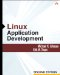# Working with User-Defined Aggregates

## Working with User-Defined Aggregates

SQL Server has aggregate functions for such things as MAX, MIN, COUNT, and SUM, but what if you want to create a new aggregate function? You can create user-defined aggregates using managed code, which means you can create a function that will be executed once for every row in your rowset, using the column of your choice.

To create a user-defined aggregate in Visual Studio .NET, simply add an Aggregate template to your project. This template consists of a structure (C# struct), not a partial class. The structure has the SqlUserDefinedAggregate attribute, which tells Visual Studio .NET how to deploy the structure. The user-defined aggregate must implement the following four methods.

• Init Executes when the aggregate is initialized. You must initialize your fields in this method because SQL Server might choose to reuse your aggregate instead of creating a new one, and this method will be called with the expectation that the aggregate will be "like new" and ready for use.

• Accumulate Called once for each row that is to be aggregated.

• Merge If SQL Server decides to use multiple threads to perform the aggregation, each thread uses its own aggregate object. The accumulated results of the objects are merged to get the combined result. Notice that Merge is handed an aggregate object, not a value, which makes it possible to access the object's member variables.

• Terminate Used to return the results.

The Format property on the SqlUserDefinedAggregate attribute tells SQL Server how to handle serialization. If all members of the class are value types, you can use Format.Native. To serialize more complex data, you must do your own serialization.

You should be careful to always test the value parameter (that is passed to the Accumulate method) for null values because SQL data types are nullable. There is also an optimizer hint that is called IsInvariantToNulls, which is a property of the SqlUserDefinedAggregate attribute that you can set to true when you don't need the Accumulate to be called with null values. In many cases, you will want to ignore nulls, but if you are counting the total number of items, you might want to use null as part of the count. For example, the COUNT aggregate that SQL Server has does not count nulls if you are performing a count on a field, but you can create an aggregate that does count the nulls.

You can also choose the data type to pass into the Accumulate method, and the type to be returned from the Terminate method.

The SqlUserDefinedAggregate attribute also contains a property called IsNullIfEmpty. If you set IsNullIfEmpty to true, the aggregate returns null when there are no values to aggregate.

An example of an aggregate is one that gets the minimum date/time and the maximum date/ time and returns the time span, as shown in the following code.Visual Basic

`Imports System Imports System.Data Imports System.Data.Sql Imports System.Data.SqlTypes Imports Microsoft.SqlServer.Server <Serializable()> _ <Microsoft.SqlServer.Server.SqlUserDefinedAggregate( _    Format.Native, IsNullIfEmpty:=True, IsInvariantToNulls:=True)> _ Public Structure DateTimeSpanVb    Private minDate As SqlDateTime    Private maxDate As SqlDateTime    Public Sub Init()       minDate = SqlDateTime.Null       maxDate = SqlDateTime.Null    End Sub    Public Sub Accumulate(ByVal value As SqlDateTime)       If value.IsNull Then Return       If ((minDate.IsNull) Or (value.CompareTo(minDate) < 0)) Then          minDate = value       End If       If ((maxDate.IsNull) Or (value.CompareTo(maxDate) > 0)) Then          maxDate = value       End If    End Sub    Public Sub Merge(ByVal Group As DateTimeSpanVb)       Accumulate(Group.minDate)       Accumulate(Group.maxDate)    End Sub    Public Function Terminate() As SqlString       If (maxDate.IsNull Or minDate.IsNull) Then          Return SqlString.Null       End If       Dim ts As TimeSpan       ts = maxDate.Value - minDate.Value       Return ts.ToString()    End Function End Structure `C#

`using System; using System.Data; using System.Data.Sql; using System.Data.SqlTypes; using Microsoft.SqlServer.Server; [Serializable] [Microsoft.SqlServer.Server.SqlUserDefinedAggregate(    Format.Native, IsNullIfEmpty=true, IsInvariantToNulls=true)] public struct DateTimeSpanCs {    private SqlDateTime minDate;    private SqlDateTime maxDate;    public void Init()    {       minDate = SqlDateTime.Null;       maxDate = SqlDateTime.Null;    }    public void Accumulate(SqlDateTime Value)    {       if (Value.IsNull) return;       if ((minDate.IsNull)||(Value.CompareTo(minDate) < 0))          minDate = Value;       if ( (maxDate.IsNull)||(Value.CompareTo(maxDate) > 0))          maxDate = Value;    }    public void Merge(DateTimeSpanCs Group)    {       Accumulate(Group.minDate);       Accumulate(Group.maxDate);    }    public SqlString Terminate()    {       if (maxDate.IsNull || minDate.IsNull)          return SqlString.Null;       TimeSpan ts;       ts=maxDate.Value - minDate.Value;      return ts.ToString();    } } `In this sample, two fields are defined: minDate and maxDate. These fields are set to null in the Init method, which ensures that these values are initialized properly even if SQL Server reuses this structure. The Accumulate method signature accepts a SqlDateTime type for the Value parameter. You can test this aggregate by adding the following script to the Test.sql file.Visual Basic

`SELECT dbo.DateTimeSpanVb(ShippedDate) AS TimeSpan    FROM orders `C#

`SELECT dbo.DateTimeSpanCs(ShippedDate) AS TimeSpan    FROM orders `The output should look like the following, which shows 665 days between the first ship date and the last ship date.Output Window: User-Defined Aggregate

`TimeSpan ----------------------- 665.00:00:00 No rows affected. (1 row(s) returned) `Sometimes you want to manually register the aggregate in SQL Server. The following SQL script shows the syntax.SQL: User-Defined Aggregate Registration

`CREATE AGGREGATE <user defined aggregate name> (    @Value <data type of value> ) RETURNS <data type of return value>    EXTERNAL NAME <assembly name>.<aggregate name>' `Linux Application Development (2nd Edition)
ISBN: 735621411
EAN: 2147483647
Year: 2004
Pages: 85
Authors: Michael K. Johnson, Erik W. Troan

Similar book on Amazon